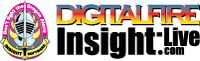•The secret to cool bodies and glazes is a lot of testing.
•The secret to know what to test is material and chemistry knowledge.
•The secret to learning from testing is documentation.
•The place to test, do the chemistry and document is an account at https://insight-live.com
•The place to get the knowledge is https://digitalfire.com

Sign-up at https://insight-live.com today.

# Clayburn Fireclay

 Oxide Analysis Formula CaO 0.39% 0.026 MgO 0.07% 0.006 K2O 1.16% 0.045 Na2O 1.16% 0.069 TiO2 1.97% 0.091 Al2O3 27.66% 1.000 SiO2 54.25% 3.329 Fe2O3 1.50% 0.035 LOI 11.84 Oxide Weight 325.10 Formula Weight 368.76

## XML for Import into INSIGHT

<?xml version="1.0" encoding="UTF-8"?> <material name="Clayburn Fireclay" descrip="" searchkey="" loi="11.84" casnumber="70694-09-6"> <oxides> <oxide symbol="CaO" name="Calcium Oxide, Calcia" status="" percent="0.390" tolerance=""/> <oxide symbol="MgO" name="Magnesium Oxide, Magnesia" status="" percent="0.070" tolerance=""/> <oxide symbol="K2O" name="Potassium Oxide" status="" percent="1.160" tolerance=""/> <oxide symbol="Na2O" name="Sodium Oxide, Soda" status="" percent="1.160" tolerance=""/> <oxide symbol="TiO2" name="Titanium Dioxide, Titania" status="" percent="1.970" tolerance=""/> <oxide symbol="Al2O3" name="Aluminum Oxide, Alumina" status="U" percent="27.660" tolerance=""/> <oxide symbol="SiO2" name="Silicon Dioxide, Silica" status="" percent="54.250" tolerance=""/> <oxide symbol="Fe2O3" name="Iron Oxide, Ferric Oxide" status="" percent="1.500" tolerance=""/> </oxides> <volatiles> <volatile symbol="LOI" name="Loss on Ignition" percent="11.840" tolerance=""/> </volatiles> </material>